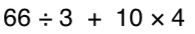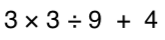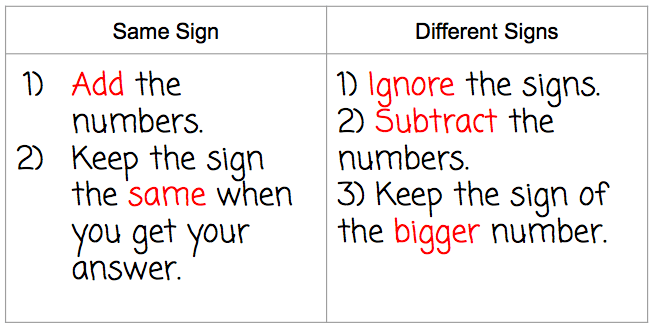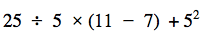Vocab
Exponents
Order of Operations
Fractions
Multiply fractions
100

the top number of a fractions is called...

Numerator

100

24

16

100

Simplify the following expression:62

100

-4 + -5

9100

simplify 4/8

1/2

100

3/4 x 1/5

3/20

200

This is made up of a whole number and fraction...

mixed number

200

What is 9^3 (exponent)

729

200

Simplify the following expression:5

200

14 + -12

2

https://www.khanacademy.org/math/arithmetic/arith-review-negative-numbers/arith-review-add-negatives-intro/v/adding-negative-numbers200
2/3 + 2 and 1/3

2

200

6/7 x 2/7

12/49

300

The bottom number of a fraction is called...

denominator

300

what is 2^5

32

300

3 + 42 * (3 + 2)

83

300

-10 + 15

5300

Can 7/20 be simplified?

No

300

2 and 4/5 x 3 and 1/3

140/15 OR 9 and 1/3

400

When the numerator is larger than the denominator

improper fraction

400

What is the value of:

84

4,096

40045

400

-18 + (-12) =

-30

400

3/4 + 1/6

11/12

400

Product

500

we do not a "reduce" a fraction, we...

simplify

500

What is the value of:

23 + 32 - 42

1

50030

500

6 + (-2 + -5)

-1

500

*Challenge* Divide the following

2/5 divided by 7/10

4/7

500

4 and 5/12 x 3 and 3/4

795/48 or 16 and 9/16

Click to zoom